View on GitHub

# Pedro Paulo Oliveira Jr

## Personal website

Studying at PUC-Rio there was a course called Probabilistic Models given by the Telecommunication Engineering Department, which was well-known for their creative approach to student evaluation. In one of those exams there was a question described below:

Suppose you break a stick in a random point of its length, from the two pieces you have now, choose the largest piece and break again randomly. What is the likelihood of you form a triangle with the three pieces?

Before you go nuts looking in Google, note that this problem is slightly different from the "three stick triangle problem" , in ours the second time you choose to break the biggest piece which is different of breaking a stick into 3 pieces directly. Warning: if you want to try to solve it, stop reading now. There are two ways to solve this problem, a computational way, that was not the case at the time, and an analytical form. The analytic form is interesting but requires further mathematical knowledge. But a computational form is available for anyone who knows how to program as in the Python script below.

 from random import random def isTriangle ( a , b , c ) : listT = [ a , b , c ] listT. sort ( ) if ( listT < (listT + listT)) : return True else : return False   def breakRule ( ) : p = random ( ) if ( p < 0.5 ) : a = p p1 = random ( ) b = ( 1 -p ) * p1 c = ( 1 -p ) - b else : a = ( 1.0 - p ) p1 = random ( ) b = p * p1 c = p - b return isTriangle ( a , b , c )   yes = 0 no = 0   for i in xrange ( 100000 ) : if breakRule ( ) : yes + = 1 else : not + = 1   print "Number of times that worked:% d" % yes print "Number of times that went wrong:% d" % not print "Probability:% f" % ( float (yes)) / (yes + no) 

Now let's see how to solve it analytically. <continue> Considering the ruler as having length 1, we divide the problem into two parts that are symmetrical. In the first part the break occurred at the point n ( 0 <n <0.5 ) until 50% of the length of the ruler. Thus the second break may not occur, to respect the triangular inequality:

$latex A>B+C$

where A is the length of the longest piece in the sections:

$latex [N,\frac{1}{2}];[1-(\frac{1}{2}-n);1]$

As you can see in the figure below: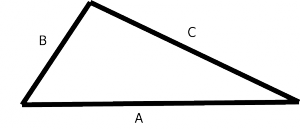Thus, once the break point in the ruler n>0.5  the probability of forming a triangle is given by: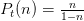Therefore, the probability of forming a triangle is: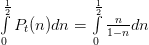Solving the integral we get: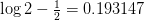As we were two symmetrical that the probability of forming a triangle problems is: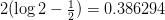That's the same value obtained by our simulation.